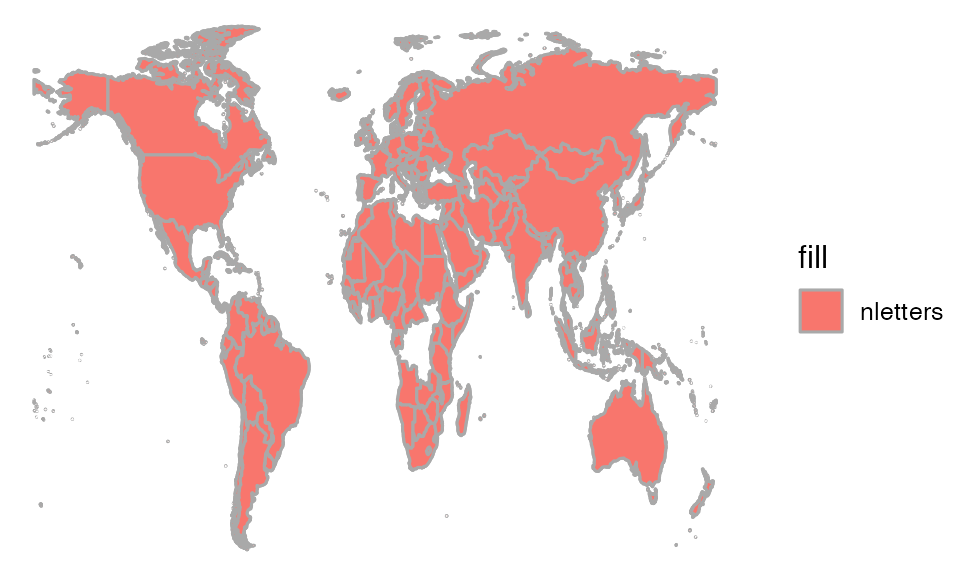This vignette is simply a suite of plots that exist primarily as part of our quality control for the package. But since the examples might be useful to others as well, we’ve added this as a vignette in the package.

This way of doing this is largely superceded by our ggformula package which provides a formula interface to ggplot2. You might also like to see the vignette that compares using lattice to using ggformula.

### lattice extras

The mosaic package resets the default panel function for histograms. This changes the default for bin selection and provides some additional arguments to histogram.

histogram(~ rbinom( 500, 20, .3), width=1, fit="normal", v=c(6,10), h=0.1 )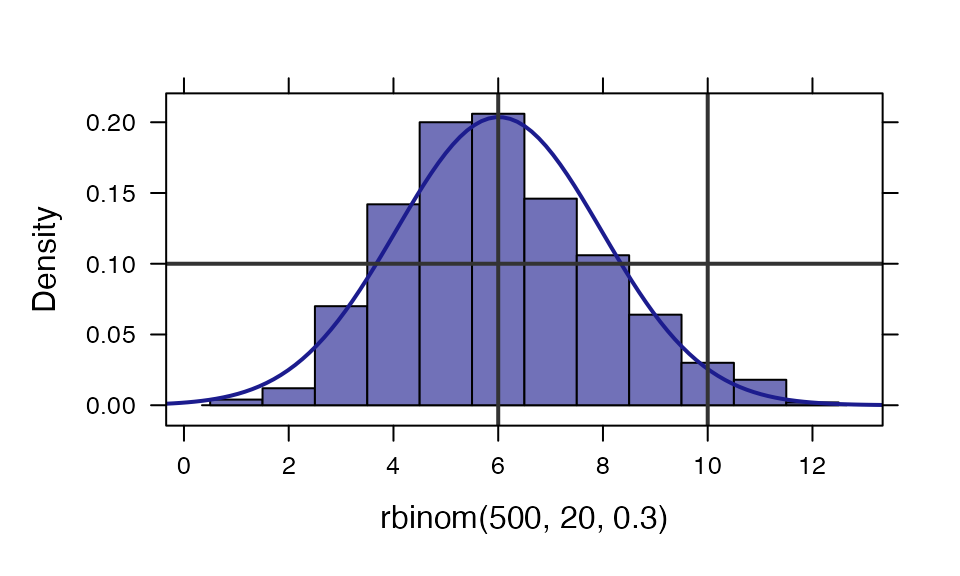ladd() provides a relatively easy way to add additional things to a lattice graphic.

xyplot( rnorm(100) ~ rnorm(100) )
ladd( grid.text("Here is some text", x=0, y=0, default.units="native") )
ladd( panel.abline( a=0, b=1, col="red", lwd=3, alpha=.4 ) )
ladd( panel.rect(x=-1, y=-1, width=1, height=1, col="gray80", fill="lightsalmon"))
ladd( panel.rect(x=0, y=0, width=2, height=2, col="gray80", fill="lightskyblue"),
under=TRUE)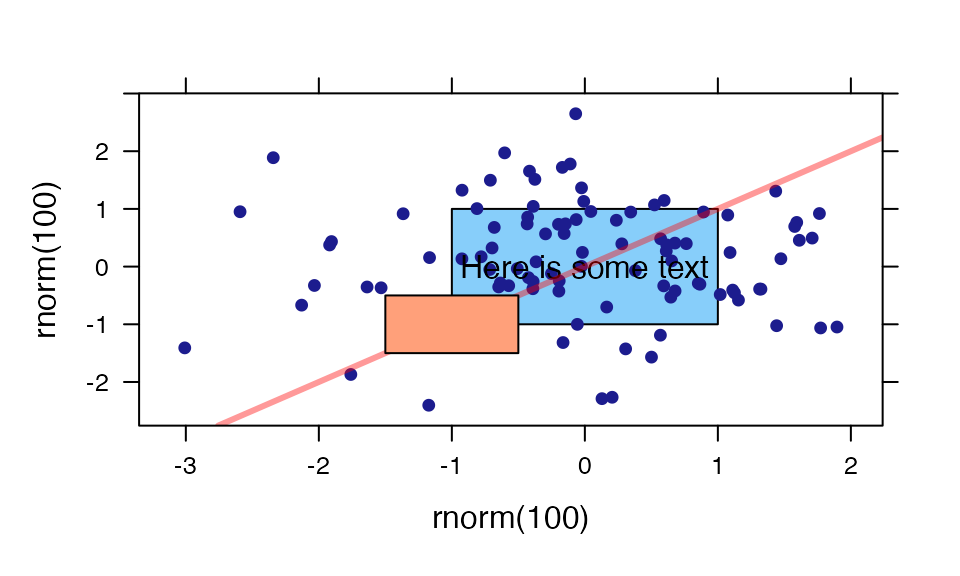### mplot()

In addition to the interactive uses of mplot(), it can be used in place of plot() in several settings.

require(gridExtra)
mod <- lm(width ~ length * sex, data = KidsFeet)
mplot(mod, which = 1:7, multiplot = TRUE, ncol = 2)
## geom_smooth() using formula = 'y ~ x'
## geom_smooth() using formula = 'y ~ x'
## geom_smooth() using formula = 'y ~ x'
## geom_smooth() using formula = 'y ~ x'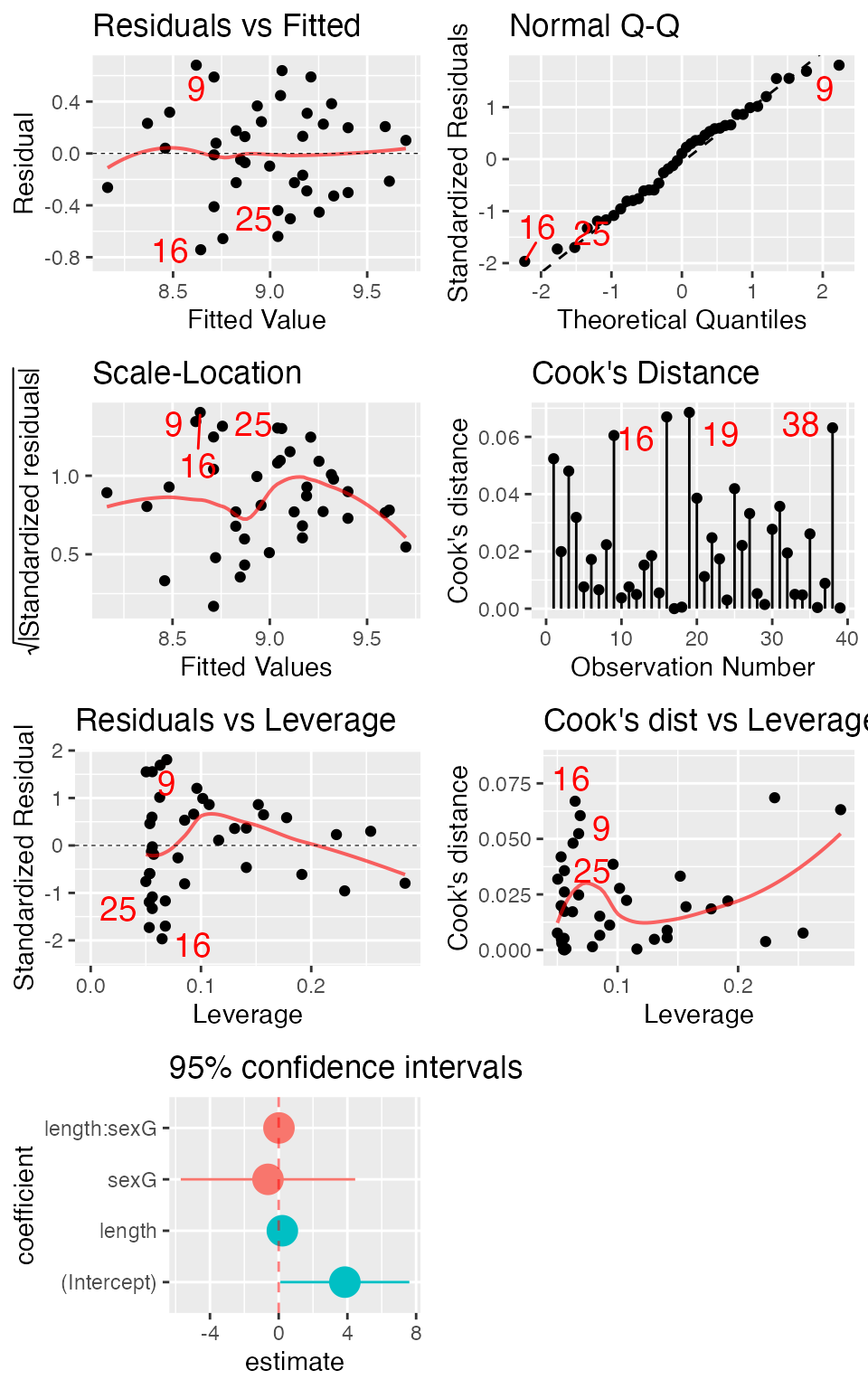mplot(mod, which=1:7, system="ggplot", ncol=2)
## geom_smooth() using formula = 'y ~ x'
## geom_smooth() using formula = 'y ~ x'
## geom_smooth() using formula = 'y ~ x'
## geom_smooth() using formula = 'y ~ x'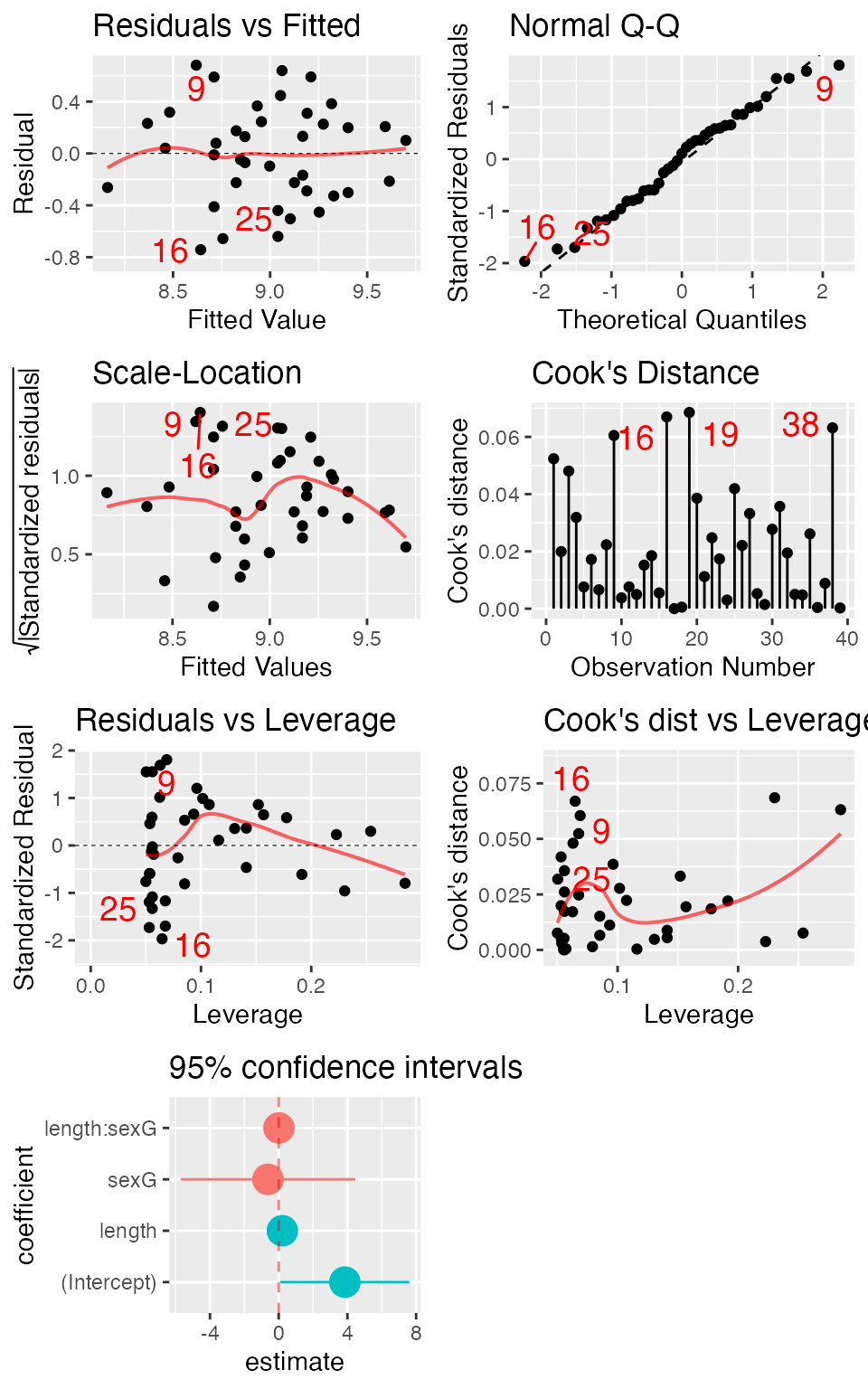mplot(mod, which=7)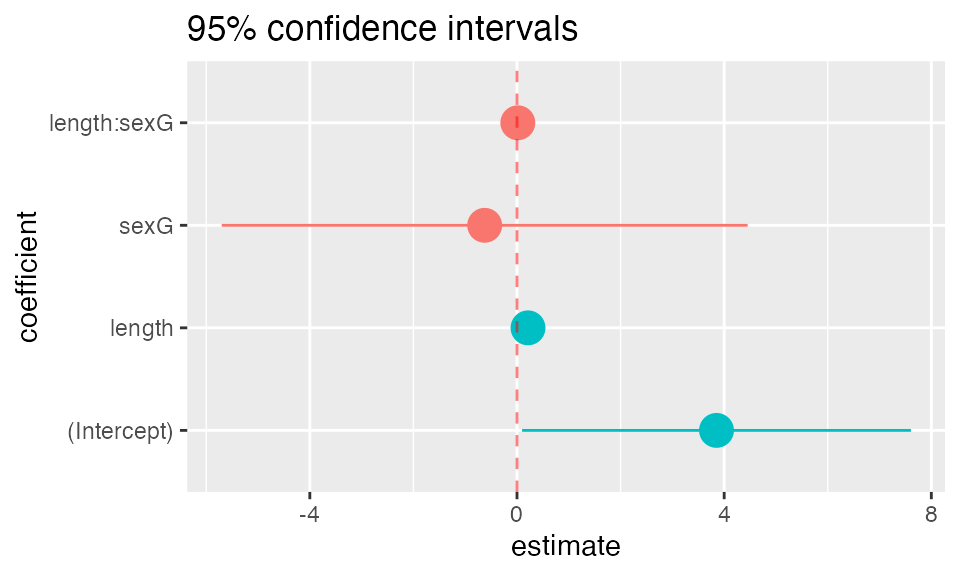mplot(mod, which=7, rows=-1)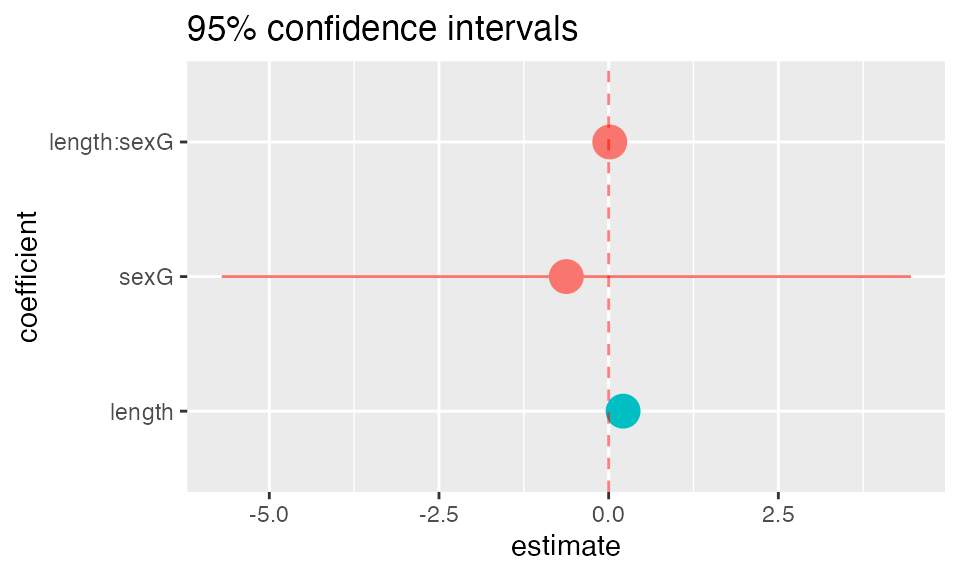mplot(mod, which=7, rows=c("sexG", "length", "length:sexG"),
title="Custom titles are supported")mod <- lm(age ~ substance, data=HELPrct)
mplot(TukeyHSD(mod))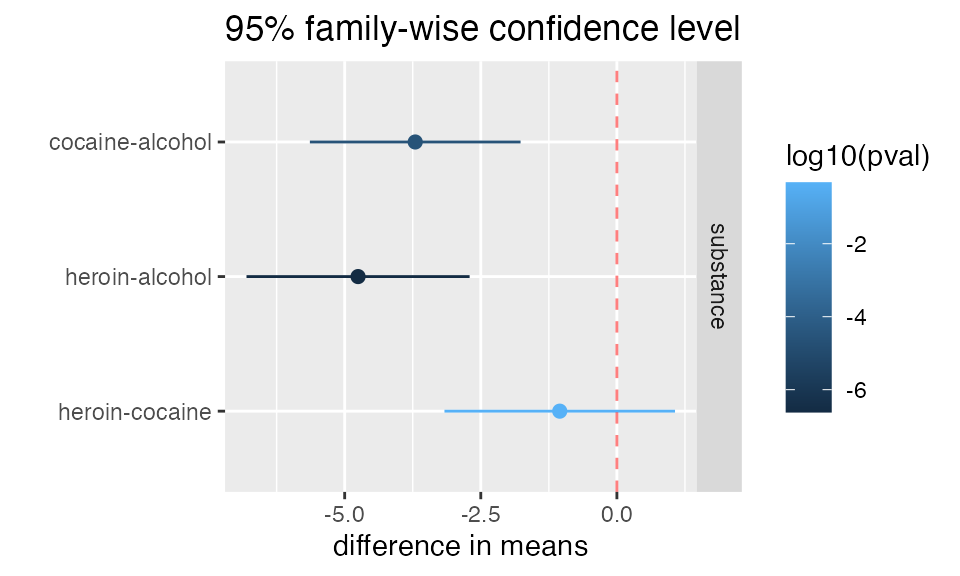mplot(TukeyHSD(mod), system="ggplot")### plotFun() and makeFun()

mod <- lm(width ~ length* sex, data = KidsFeet)
L <- makeFun(mod)
L( length=15, sex="B")
##        1
## 7.041437
L( length=15, sex="G")
##        1
## 6.654868
xyplot(width ~ length, groups = sex, data = KidsFeet, auto.key=TRUE)
plotFun( L(length, sex="B") ~ length, add=TRUE, col=1 )
## converting numerical color value into a color using lattice settings
plotFun( L(length, sex="G") ~ length, add=TRUE, col=2 )
## converting numerical color value into a color using lattice settings
## converting numerical color value into a color using lattice settings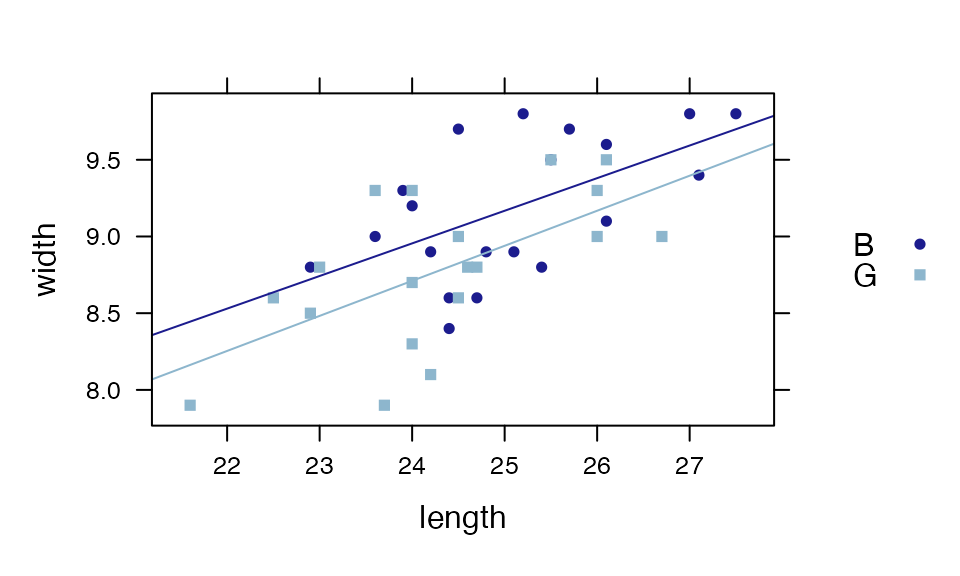For logistic regression, makeFun() handles the conversion back to probabilities by default.

mod <- glm( SmokeNow =="Yes" ~ Age + Race3, data=NHANES, family=binomial())
SmokerProb <- makeFun(mod)
xyplot( SmokeNow=="Yes" ~ Age, groups=Race3, data=NHANES, alpha=.01, xlim=c(20,90) )
plotFun(SmokerProb(Age, Race3="Black") ~ Age, col="black", add=TRUE)
plotFun(SmokerProb(Age, Race3="White") ~ Age, col="red", add=TRUE)
ladd(grid.text("Black", x=25, y=SmokerProb(25, Race="Black"),hjust = 0, vjust=-0.2,
gp=gpar(col="black"),
default.units="native"))
ladd(grid.text("White", x=25, y=SmokerProb(25, Race="White"),hjust = 0, vjust=-0.2,
gp=gpar(col="red"),
default.units="native"))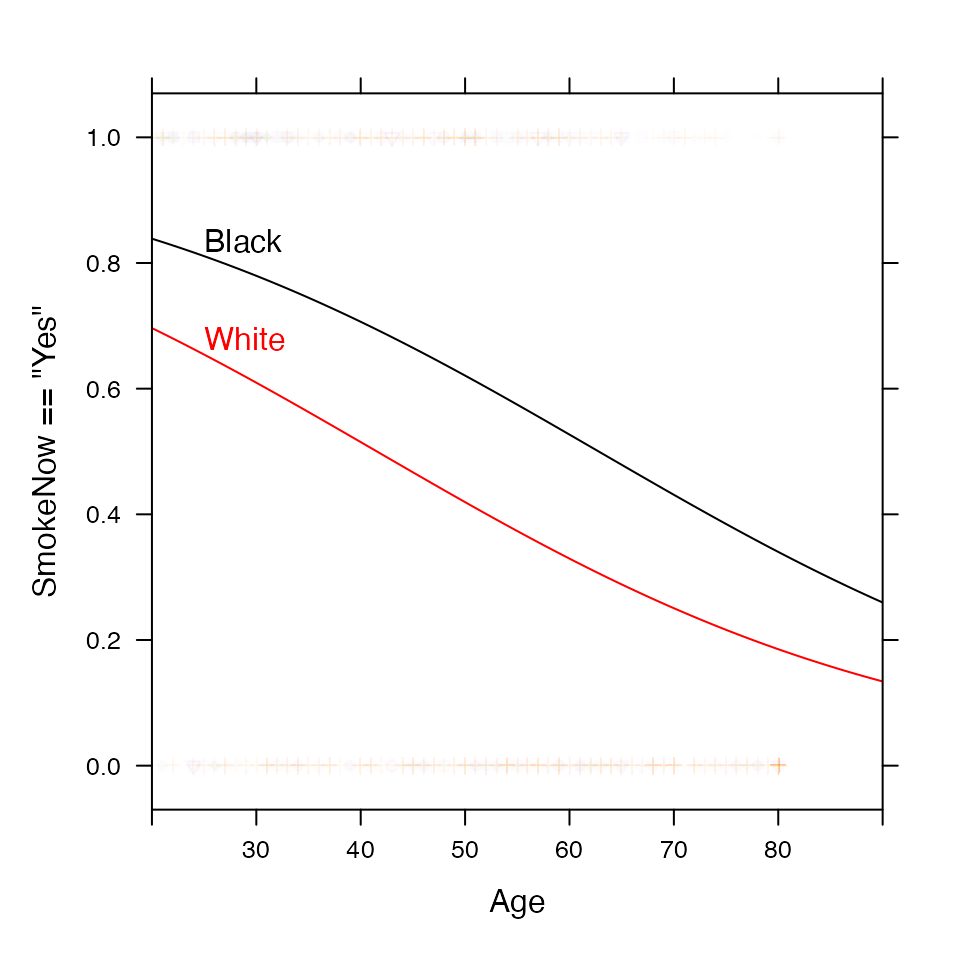f <- makeFun(sin(x) ~ x)
plotFun( f(x) ~ x, xlim = c( -2 * pi, 2 * pi) )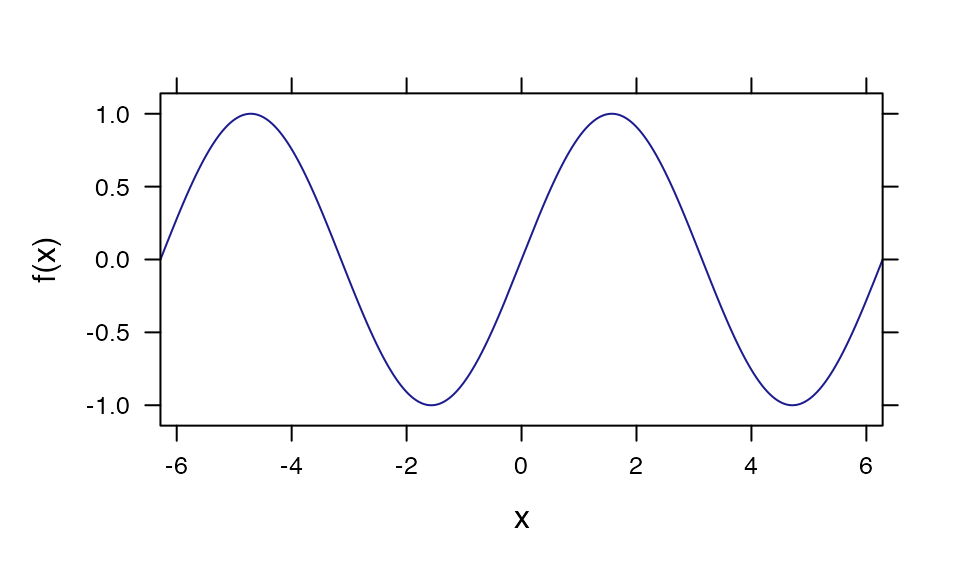plotFun( x * sin(1/x) ~ x, xlim=c(-1,1) )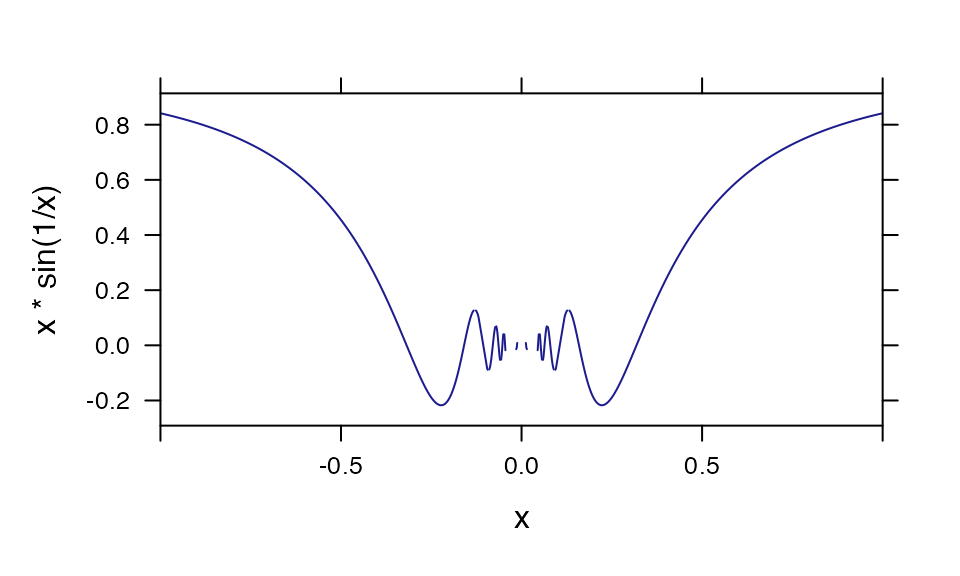plotFun( x * sin(1/x) ~ x, xlim=c(-1,1), npts=10000 )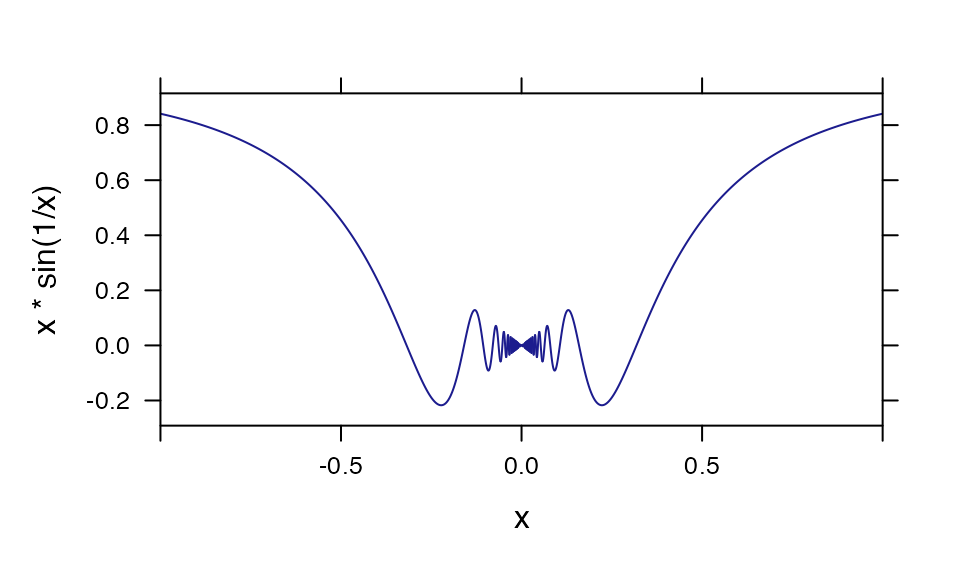### Visualizing distributions

plotDist("chisq", df=3)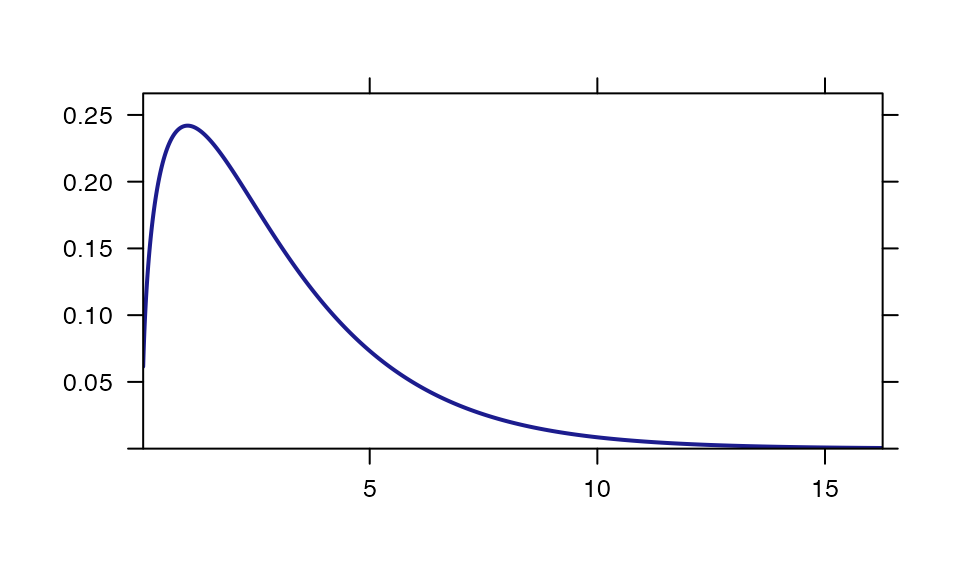plotDist("chisq", df=3, kind="cdf")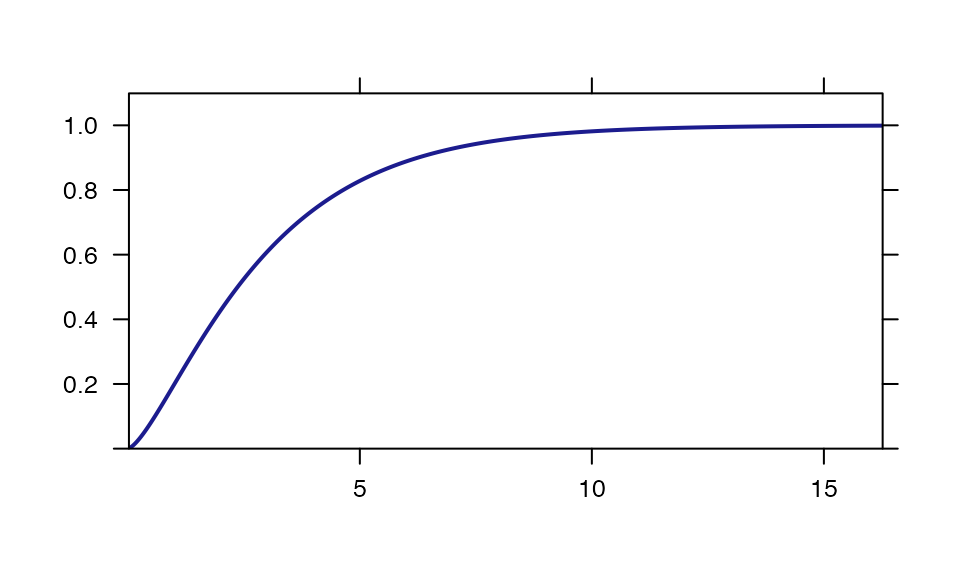xpnorm(80, mean=100, sd=15)
## 
## If X ~ N(100, 15), then
##  P(X <= 80) = P(Z <= -1.333) = 0.09121
##  P(X >  80) = P(Z >  -1.333) = 0.9088
##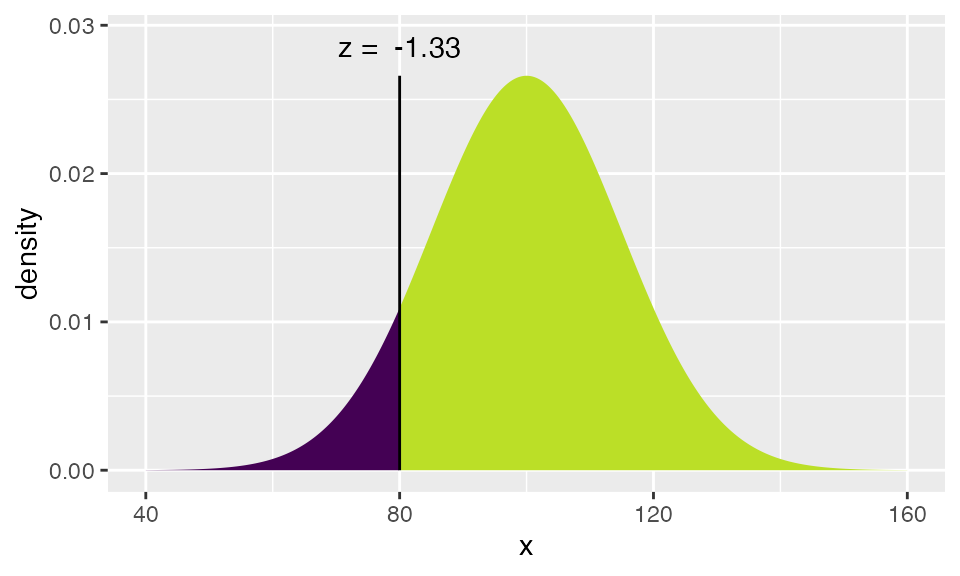##  0.09121122
xpnorm(c(80,120), mean=100, sd=15)
## 
## If X ~ N(100, 15), then
##  P(X <=  80) = P(Z <= -1.333) = 0.09121  P(X <= 120) = P(Z <=  1.333) = 0.90879
##  P(X >   80) = P(Z >  -1.333) = 0.90879  P(X >  120) = P(Z >   1.333) = 0.09121
##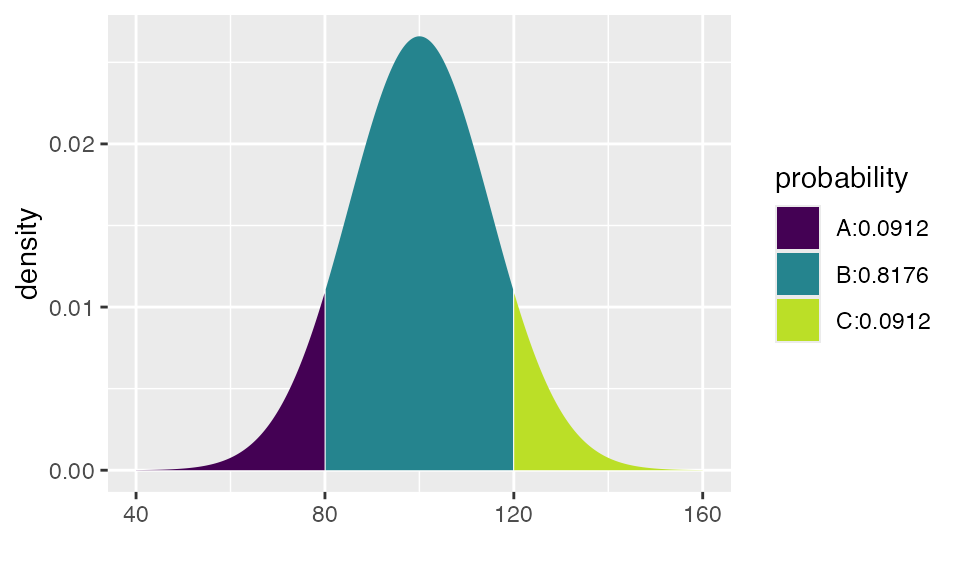##  0.09121122 0.90878878
pdist("chisq", 4, df=3)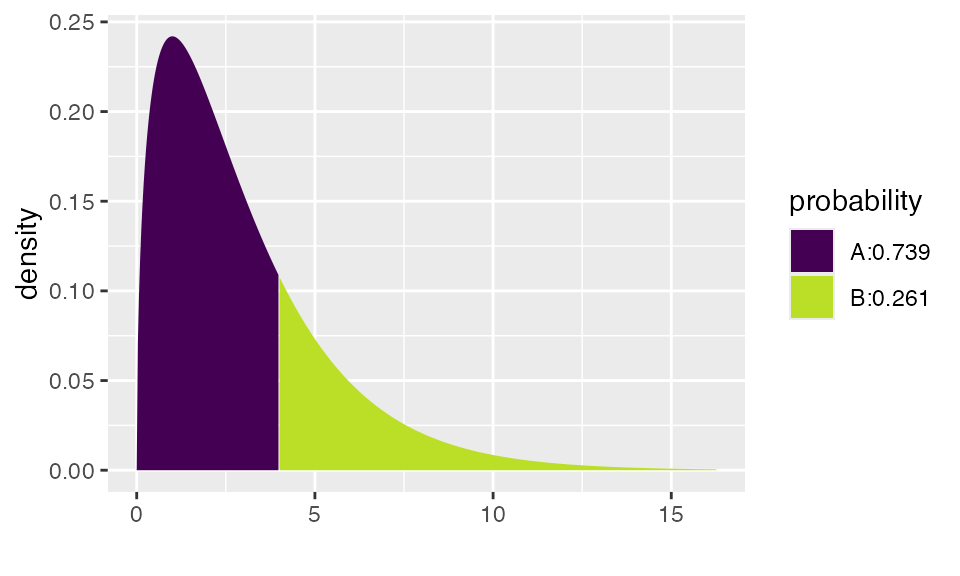##  0.7385359
pdist("f", 3, df1=5, df2=20)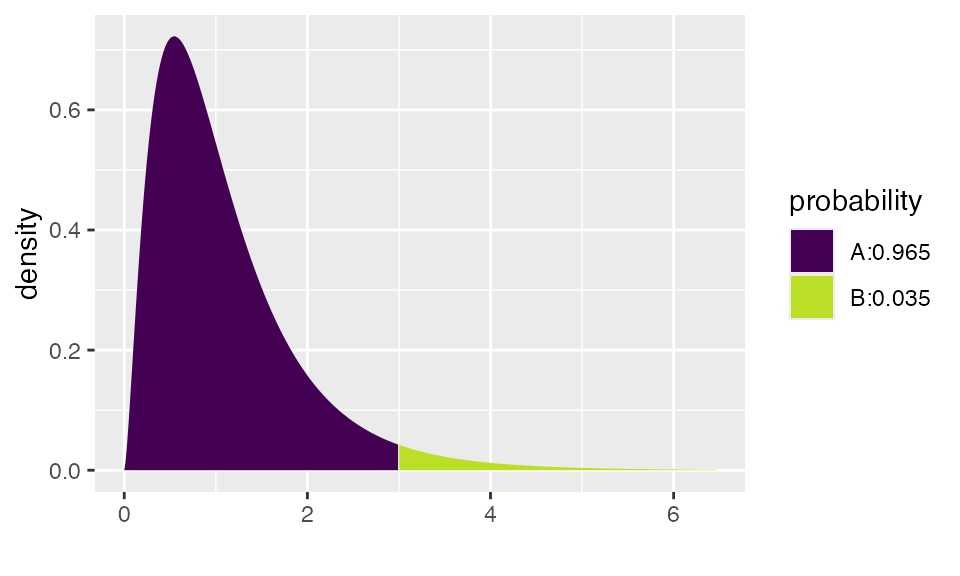##  0.9647987
qdist("t", c(.025, .975) , df=5)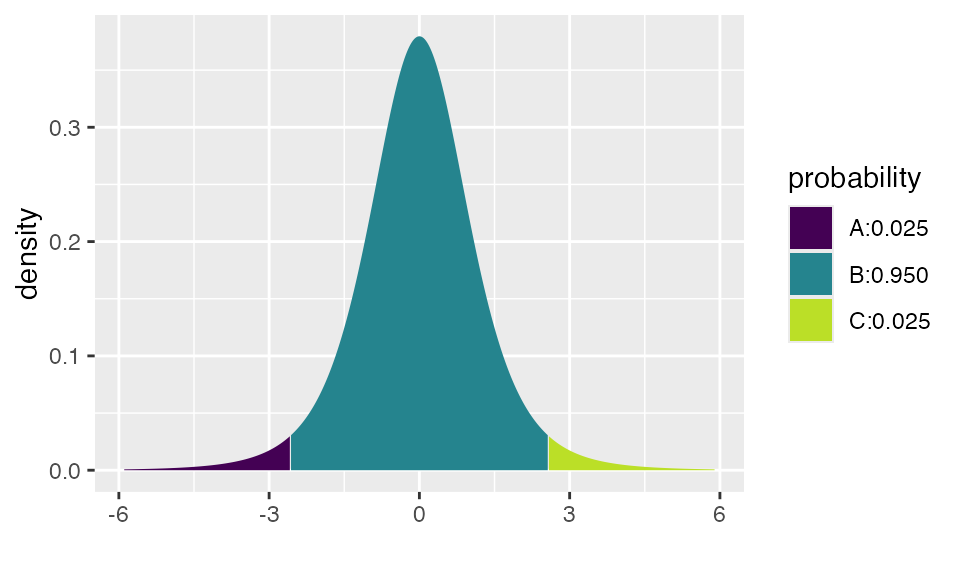##  -2.570582  2.570582
histogram( ~ rbinom(1000, 20, .4), width=1, v=20 * .4 )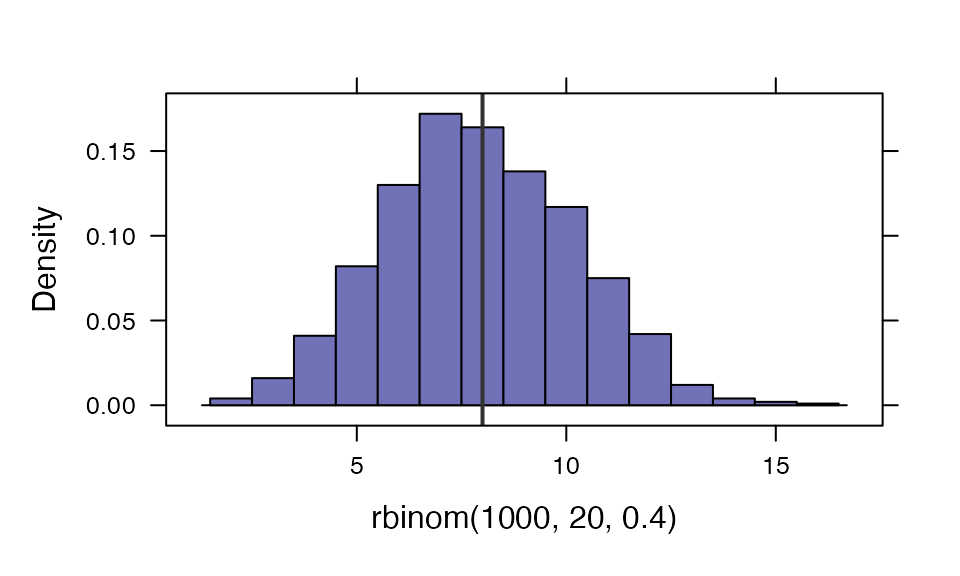SD <- sqrt(20 * .4 * .6)
plotDist("norm", mean=.4*20, sd=SD, add=TRUE, alpha=.7)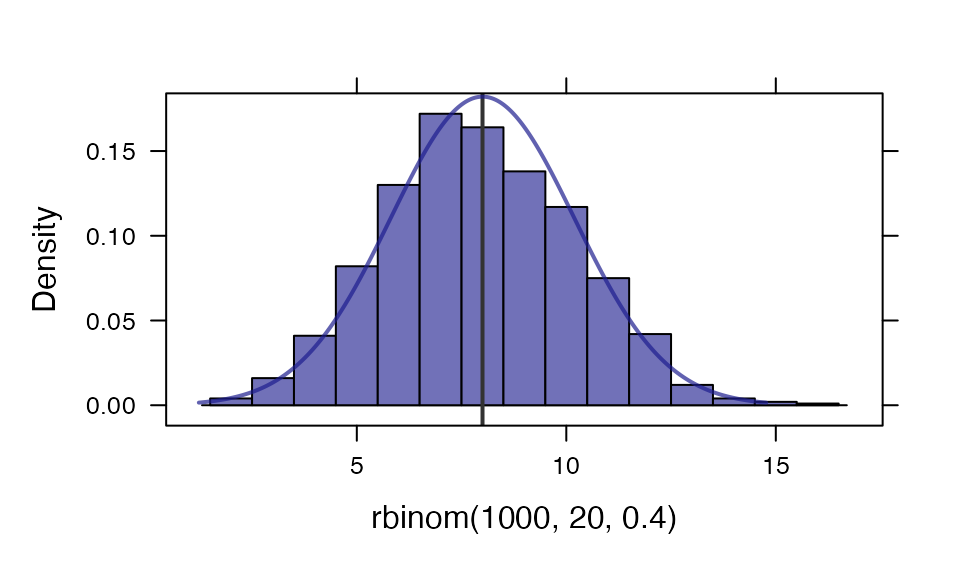plotDist("norm", col="blue", mean=2, xlim=c(-4,8))
plotDist("norm", mean=0, col="red", kind='histogram', under=TRUE)  # add, but underneath!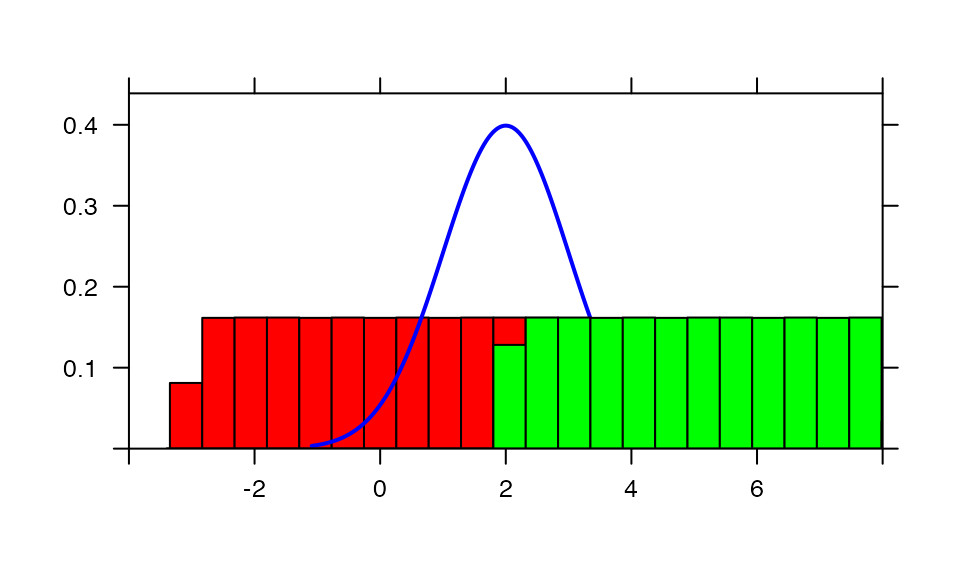### Maps

The mosaic package now provides facilities for producing choropleth maps. The API is still under developement and may change in future releases.

mUSMap(USArrests %>% mutate(state = row.names(.)), key="state", fill = "UrbanPop") 
## Mapping API still under development and may change in future releases.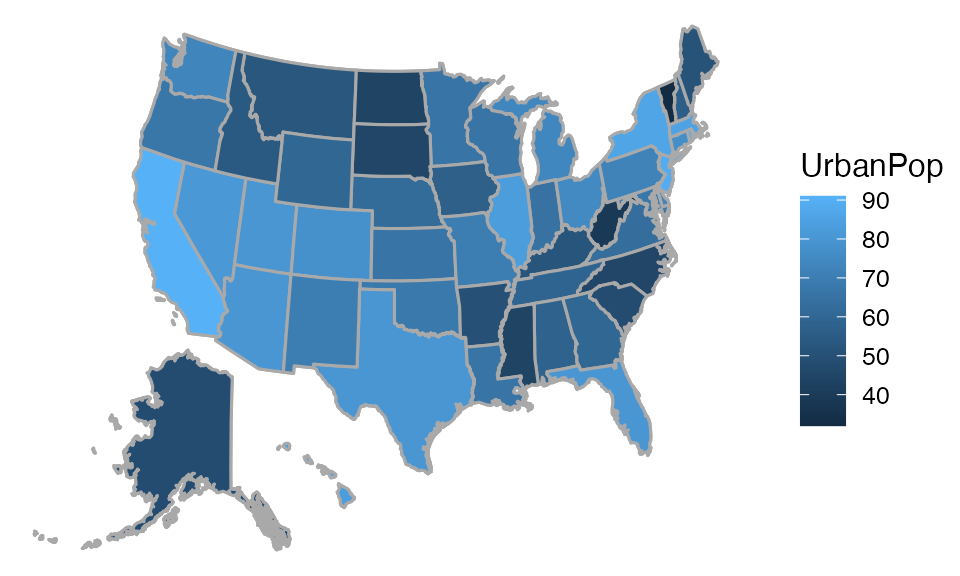Looks like it is safer to live in the North:

mUSMap(USArrests %>% mutate(state = row.names(.)), key="state", fill = "Murder") 
## Mapping API still under development and may change in future releases.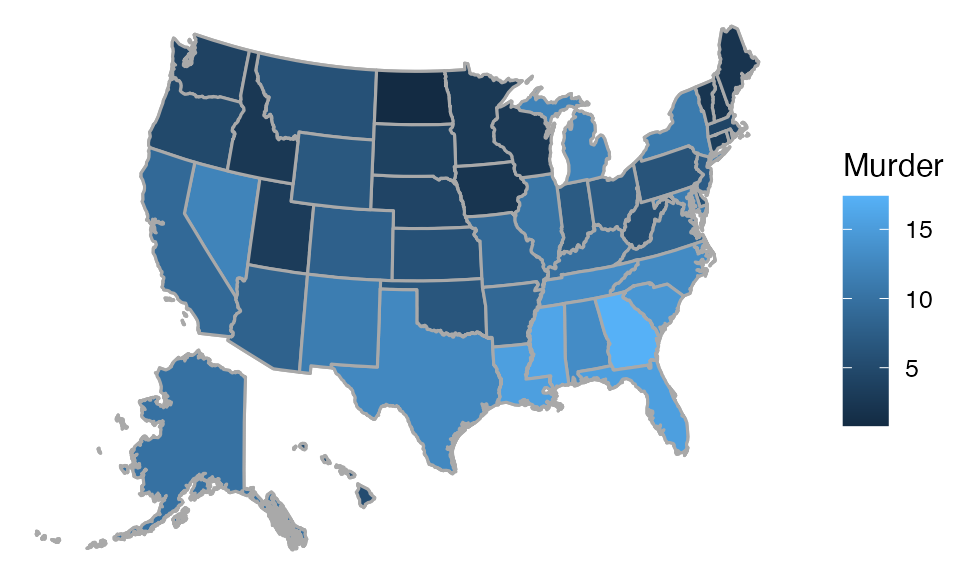Here is a sillier example

Countries %>% mutate(nletters = nchar(gapminder)) %>%
mWorldMap(key="gapminder", fill="nletters") 
## Mapping API still under development and may change in future releases.
## Warning in standardName(x, countryAlternatives, ignore.case = ignore.case, : 99
## items were not translated